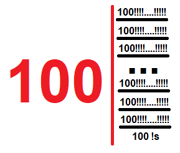11,053 PagesVisual representation of Trifactor

The Trifactor is defined as 100!3 in Extensible ! Notation. The term was coined by Yabuszko. This number is comparable to gaggol and greagol.

## Etymology

The name of this number is based on Greek prefix "tri-" and the factor.

## Approximations

Notation Approximation
Arrow notation $$82 \uparrow\uparrow\uparrow 101$$
Chained arrow notation $$82 \rightarrow 101 \rightarrow 3$$
BEAF $$\{82,101,3\}$$
Hyper-E notation $$\textrm{E}160\#100\#100$$
Hyperfactorial array notation $$100!2$$
Bird's array notation $$\{82,101,3\}$$
Strong array notation $$s(82,101,3)$$
Nested factorial notation $$100!$$
Fast-growing hierarchy $$f_4(100)$$
Hardy hierarchy $$H_{\omega^4}(100)$$
Slow-growing hierarchy $$g_{\zeta_0}(101)$$

## Sources

Community content is available under CC-BY-SA unless otherwise noted.